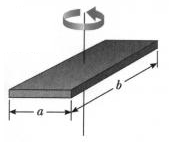Rectangular Plane Mass Moment of Inertia Calculator

Mass Moment of Inertia Equations and Calculators

 I = m (a 2 + b 2 ) / 12 Where: m = Mass (lbm , kg) a, b = short and long sides (in, mm)Solid Plate Mass Moment of Inertia Calculator Design Variables Length a (in, mm) Length b (in, mm) = m (lbs, g) = Results I (lbm-in 2, g-mm2)Membership Register | LoginHomeEngineering Book StoreEngineering ForumExcel App. DownloadsOnline Books & ManualsEngineering NewsEngineering VideosEngineering CalculatorsEngineering ToolboxGD&T Training Geometric Dimensioning TolerancingDFM DFA TrainingTraining Online EngineeringAdvertising CenterCopyright Notice